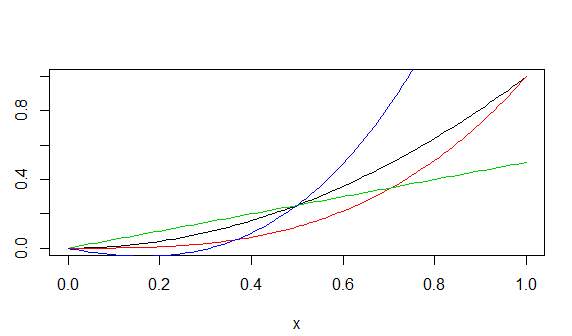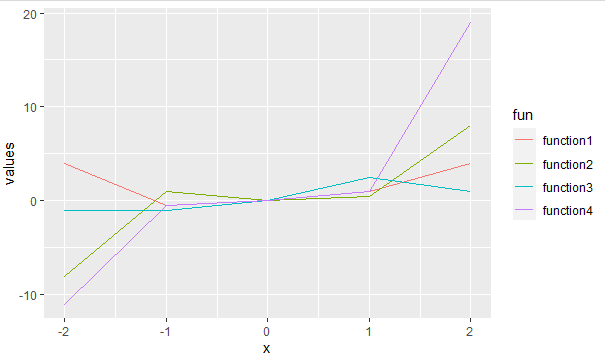# Draw Multiple Function Curves to Same Plot in R

• Last Updated : 30 May, 2021

In this article, we will discuss how to plot multiple function curves to the same plot in the R programming language.

#### Method 1: In Base R

Base R supports a function curve() which can be used to visualize a required function curve. It supports various parameters to edit the curve according to requirements.

Syntax: curve(expression, to, from, col)

Parameters:

• expression: To be curved
• to, from: range of curve plotting
• col: color of curve

To draw multiple curves in one plot, different functions are created separately and the curve() function is called repeatedly for each curve function. The call for every other curve() function except for the first one should have added an attribute set to TRUE so that multiple curves can be added to the same plot. To differentiate among the different colors are used.

Example:

## R

 `function1<- ``function``(x){x ** 2}``function2<-``function``(x){x ** 3}``function3<-``function``(x){x / 2}``function4<-``function``(x){2*(x ** 3)+(x ** 2)-(x / 2)}`` ` `curve``(function1, col = 1)``curve``(function2, col = 2, add = ``TRUE``)``curve``(function3, col = 3, add = ``TRUE``)``curve``(function4, col = 4, add = ``TRUE``)`

Output:#### Method 2: Using ggplot

GGPLOT2 is an R library used to visualize plots with its various easy-to-use functions. To draw multiple curves using gglot functions are first created normally. But to draw them in the same plot, the functions are converted to dataframe and then visualized.

Example:

## R

 `library``(``"ggplot2"``)``function1<- ``function``(x){x ** 2}``function2<-``function``(x){x ** 3}``function3<-``function``(x){x / 2}``function4<-``function``(x){2*(x ** 3)+(x ** 2)-(x / 2)}`` ` `df=``data.frame``(x = -2 : 2,``              ``values = ``c``(``function1``(-2 : 2),``                       ``function2``(-2 : 2),``                       ``function3``(-2 : 2),``                       ``function4``(-2 : 2)),``              ``fun = ``rep``(``c``(``"function1"``, ``"function2"``,``                          ``"function3"``, ``"function4"``))``              ``)`` ` `ggplot``(df, ``aes``(x, values, col = fun))+``geom_line``()`

Output:My Personal Notes arrow_drop_up